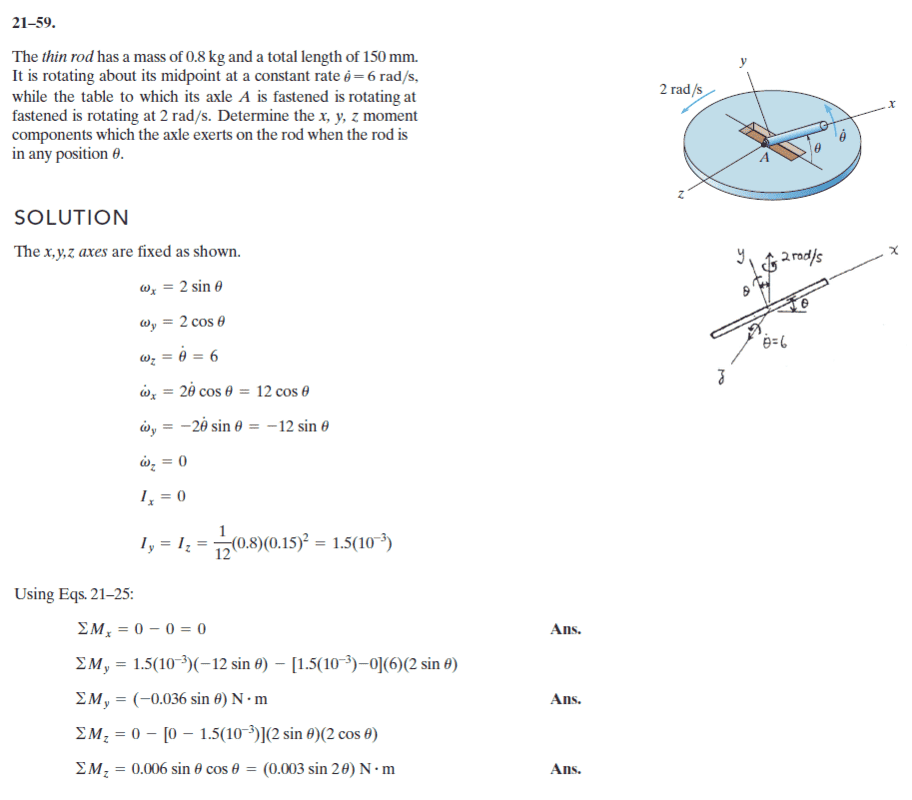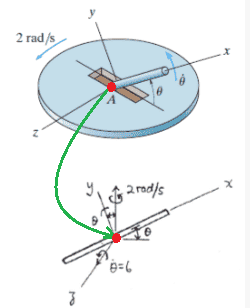# Calculating the Moment from a Different Vantage Point

• Tymofei

#### Tymofei

Homework Statement
Dynamics
Relevant Equations
General moment eq
Summary:: Just a simple 3d rigid dynamics question which I am trying to solve by placing coordinat system differently from original solution.Everything looks ok but results are different.Mod note: Post moved from technical section.
Thats my question.As you see coordinate system was located at center of mass.I just shifted it to point A and recalculated values.
Everything was same except Inertia about z and y-axis which was multiplied by 4.But at the same time moment effect coming from gravitational force was included to calculations so i thought they will cancel each other.But results was different than was mentioned on the original solution.
I know it looks nonsense trying to figure it when i already have solution but I am kind of obsessed .

By the way my values were:

My values for coordinate system placed at point 'A':

I_z = I_y = 6*10^-3 , I_x = I_xy = I_xz = I_yz = 0

Sum M_x = 0 = M_A_x

Sum M_y = 6*10^-3 * -12sin(theta)* -6*10^-3 * 12sin(theta) = -144* sin(theta)*10^-3 = M_A_y

Sum M_z = 6 * 10^-3 * 2 sin(2theta) = M_A_z - 0.8*9.81*0.075*sin(theta) ==> M_A_z = 6 * 10^-3 * 2 sin(2theta) + 0.8*9.81*0.075*sin(theta)

Last edited by a moderator:
•Delta2

Thats my question.As you see coordinate system was located at center of mass.I just shifted it to point A and recalculated values.
I could be wrong, but I think that the center of mass of the rod is located at point ##A##. The rod passes through the slot in the table so that you see only half of the rod in the figure. Hope I'm not misinterpreting the problem statement.

•Delta2
I could be wrong, but I think that the center of mass of the rod is located at point ##A##. The rod passes through the slot in the table so that you see only half of the rod in the figure. Hope I'm not misinterpreting the problem statement.
I don't thinks so,because in original solution it was placed at the center of rod,and inertia was calculated as '1/12*m*l^2' which gives you inertia about center of mass.

But isn't point ##A## at the center of the rod?•Tymofei, Delta2, PhDeezNutz and 1 other person
But isn't point ##A## at the center of the rod?
View attachment 262907

I didn't think so,but i guess you are right.
Thats the only way which explains .. thanks

•PhDeezNutz